# ML – Attention mechanism

• Last Updated : 16 Jul, 2020

Introduction:
Assuming that we are already aware of how vanilla Seq2Seq or Encoder-Decoder models work, let us focus on how to further take it up a notch and improve the accuracy of our predictions. We’ll consider the good old example of Machine Translation.

Motivation:
In a Seq2Seq model, the encoder reads the input sentence once and encodes it. At each time step, the decoder uses this embedding and produces an output. But humans don’t translate a sentence like this. We don’t memorize the input and try to recreate it, we are likely to forget certain words if we do so. Also, is the entire sentence important at every time step, while producing every word? No. Only certain words are important. Ideally, we need to feed in only relevant information (encoding of relevant words) to the decoder.
“Learn to pay attention only to certain important parts of the sentence.”

Attention reader! Don’t stop learning now. Get hold of all the important Machine Learning Concepts with the Machine Learning Foundation Course at a student-friendly price and become industry ready.

Goal:
Our goal is to come up with a probability distribution, which says, at each time step, how much importance or attention should be paid to the input words.

How does it works:
Consider the following Encoder- Decoder architecture with Attention.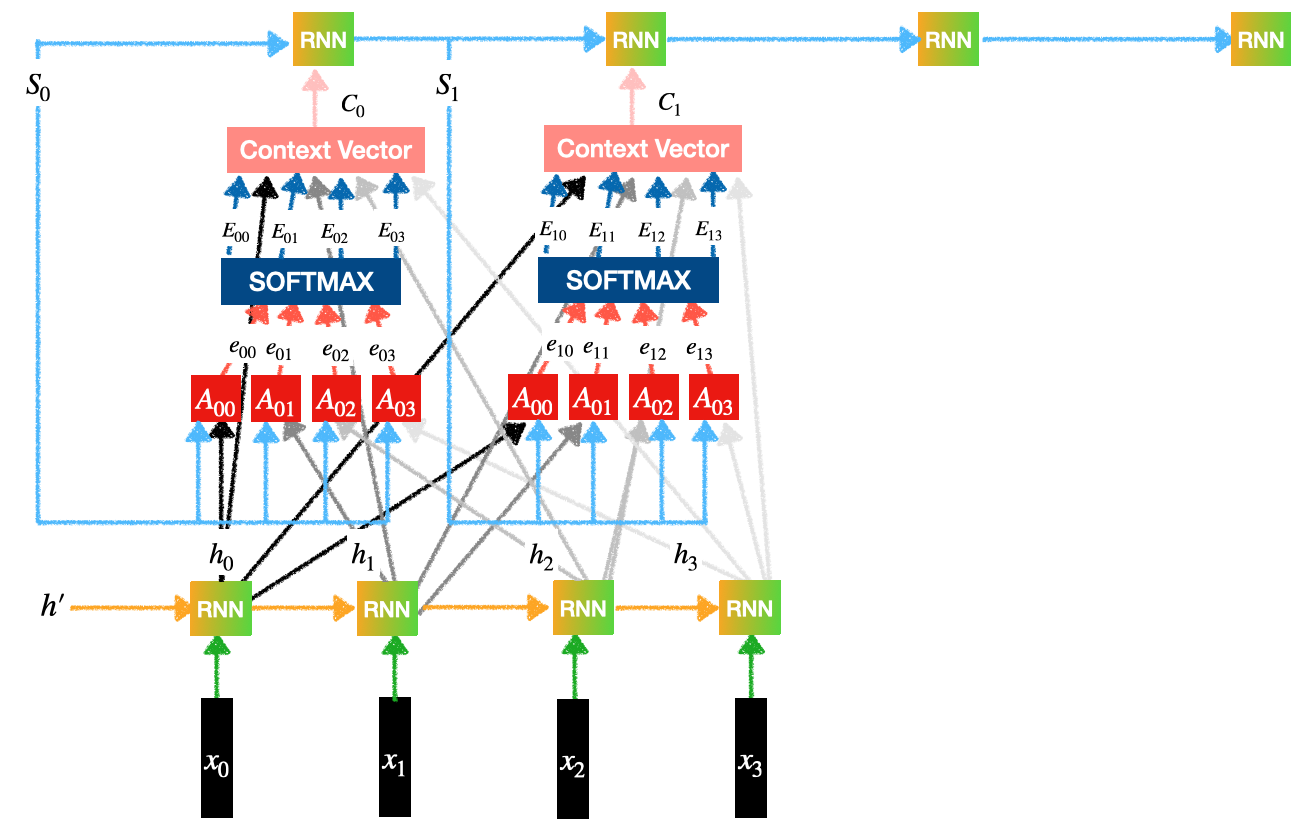Encoder-Decoder with Attention

We can observer 3 sub-parts/ components in the above diagram:

• Encoder
• Attention
• Decoder

Encoder: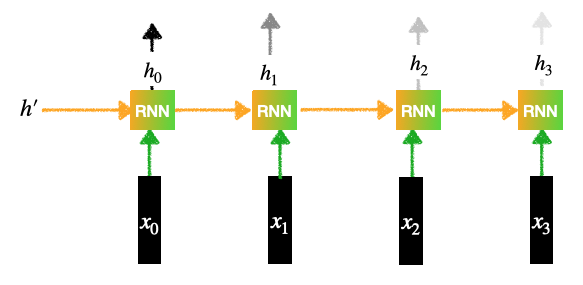Encoder

Contains a RNN layer (Can be LSTMs or GRU):
1. There are 4 inputs:2. Each input goes through an Embedding Layer.
3. Each of the input generates a hidden representation.
4. This generates the outputs for the Encoder:Attention:

• Our goal is to generate the context vectors.
• For example, context vectortells us how much importance/ attention should be given the inputs:.
• This layer in turn contains 3 sub parts:
• Feed Forward Network
• Softmax Calculation
• Context vector generation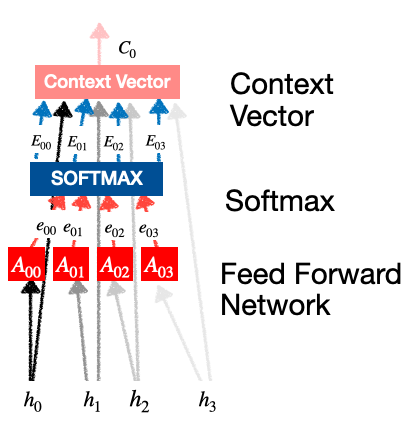attention

Feed Forward Network: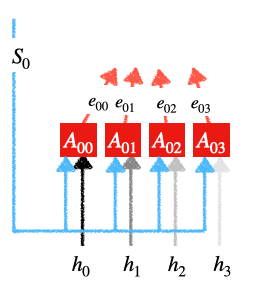Feed-Forward-Network

Eachis a simple feed forward neural network with one hidden layer. The input for this feed forward network is:
• Previous Decoder state
• Output of Encoder states.

Each unit generates outputs:..can be any activation function such as sigmoid, tanh or ReLu.

Softmax Calculation: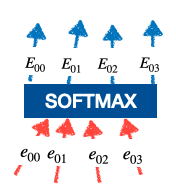softmax calculationTheseare called the attention weights. This is what that decides how much importance should be given to the inputs.

Contect Vector Generation: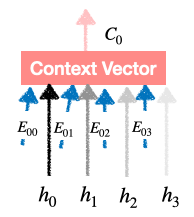context vector generation.
We findin the same way and feed it to different RNN units of the Decoder layer.

So this is the final vector which is the product of (Probability Distribution) and (Encoder’s output) which is nothing but the attention paid to the input words.
Decoder:
We feed these Context Vectors to the RNNs of the Decoder layer. Each decoder produces an output which is the translation for the input words.

Observation:
If we know the true attention weights,‘s then it would have been easier to compute the error and then adjust the parameters to minimise this loss. But in practice, we will not have this. We need someone to manually annotate each word to a set of contributing words. That is not possible.

Then why should this model work?
This is a better model when compared to the others because we are asking the model to make an informed choice. Given enough data, the model should be able to learn these attention weights just as humans do. And indeed these work better than the vanilla Encoder-Decoder models.

My Personal Notes arrow_drop_up